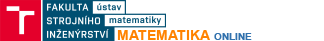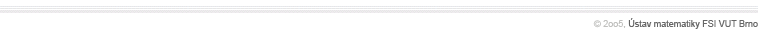3D SurfacesParametric equations of a 3D-surface, simple 3D surfaces, closed 3D surfaces, tangent plane to a surface, normal to a surface, area of a surface, Schwarzt's example, orientating a surface, orientable surfacesSurface integralSurface integral in a scalar field, definition, basic properties, and method of calculation, surface integral in a vector field, definition, basic properties, and method of calculation Operators in scalar and vector fieldsGradient of a scalar field, level lines, level surfaces, directional derivatives, vector fields, vector lines, flux through a surface, divergence of a vector field, solenoidal vector fields, Gauss-Ostrogradski theorem, curl of a vector field, irrotational vector fields, Stokes formula